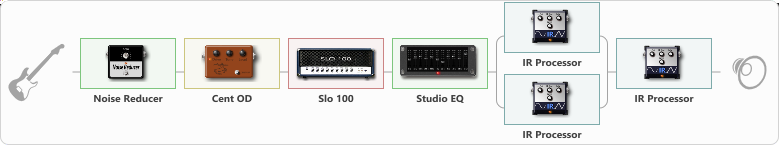# Metal - Slo100 +IR Catharsis

Discussion in 'ToneLib-GFX presets' started by Toca mal muy mal, May 24, 2021.

1. ### Toca mal muy malNew Member

Metal - Slo100 +IR Catharsis

Preset name: Slo100 IR Catharsis

Effects chain:Effect: "Noise Reducer" (Dinámica / Filtro), active - "yes"
{
"Sens" = 73
"Mode" = Soft
}

Effect: "Cent OD" (Overdrive / Distorsión), active - "yes"
{
"Drive" = 0
"Tone" = 76
"Level" = 90
}

{
"Gain" = 66
"Bass" = 60
"Middle" = 60
"Treble" = 56
"Presence" = 61
"Master" = 100
"Level (dB)" = 0
}

Effect: "Studio EQ" (Dinámica / Filtro), active - "yes"
{
"31 Hz" = 0
"62 Hz" = -15
"125 Hz" = 0
"250 Hz" = 0
"500 Hz" = 0
"1 kHz" = 1
"2 kHz" = 1
"4 kHz" = 1
"8 kHz" = 2
"16 kHz" = 0
"Level (dB)" = 2
}

Effect: "Splitter" (Dinámica / Filtro)
{
"A-Bypass" = Off
"A-Pan" = 0
"A-Level" = 55
"B-Bypass" = Off
"B-Pan" = 0
"B-Level" = 55

'A' branch:
{

Effect: "IR Processor" (Gabinetes), active - "yes"
{
"IR" = s-pres5
"Low Cut (Hz)" = 0
"Hi Cut (kHz)" = 20.0
"Mix" = 100
"Level (dB)" = 0
}
}
'B' branch:
{

Effect: "IR Processor" (Gabinetes), active - "yes"
{
"IR" = 1on-pres5
"Low Cut (Hz)" = 0
"Hi Cut (kHz)" = 20.0
"Mix" = 100
"Level (dB)" = 0
}
}
}

Effect: "IR Processor" (Gabinetes), active - "yes"
{
"IR" = Trig Room
"Low Cut (Hz)" = 0
"Hi Cut (kHz)" = 20.0
"Mix" = 2
"Level (dB)" = 20
}

Note: You will need to download and install the ToneLib-GFX software to use the preset.

File size:
28.1 KB
Views:
2,726